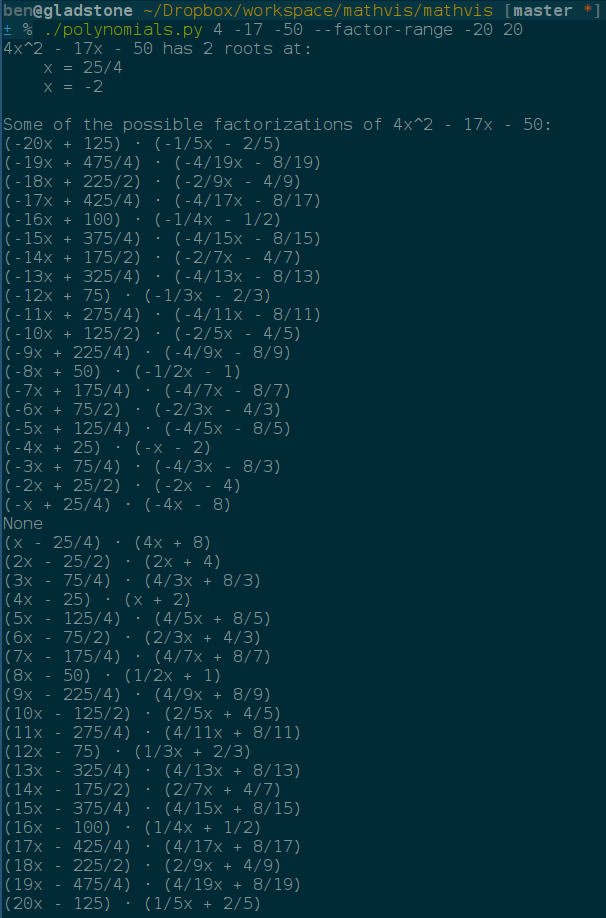polynomials.py was developed to aid in factoring quadratics when working with my students, and also to demonstrate to them that all quadratics can be factored an infinite number of ways, even the ones with complex and/or irrational roots.

From there, I've added the ability to factor higher order polynomials. It can factor any degree of polynomial as long as the polynomial has no more than 2 complex or irrational roots. So far it cannot handle polynomials with more than 2 complex or irrational roots, but this ability may be added in a future update.

Here the tool is seen factoring the quadratic x2 + 5x + 6. Notice that 8 different factorizations are generated. This is the default but it can be directed to generate as many or as few as the user likes.The tool will automatically convert decimal coefficients to fractions and use the fractions internally as long as it can, unless an irrational number is needed, in which case the tool will fall back to floating point values instead of fractions. Notice that the 6.25 passed in is converted to 25/4 before the calculations begin.Here is a quadratic with real roots being factored, but this time I have directed the tool to produce factorizations beginning with coefficients ranging from -20 to 20. The "None" in the middle is what happens when it tries to generate a factorization with a coefficient of 0.Here you can see the tool factoring a quadratic with complex roots. Notice that it finds the correct complex factors and represents the complex numbers with rational real and imaginary components. Again, this will happen automatically unless irrational numbers are needed, in which case the tool will use floating point values instead.Finally, here are a few examples of the tool factoring higher order polynomials. Currently it can only factor higher order polynomials that have no more than 2 complex or irrational roots. In this screenshot all of the polynomials have only rational roots, although the tool can handle up to 2 that are not.